HF: Hartree–Fock Theory¶

Code author: Justin M. Turney, Robert M. Parrish, and Andrew C. Simmonett

Section author: Robert M. Parrish and Jerome F. Gonthier

Introduction¶

Self-Consistent-Field (SCF) theory forms the cornerstone of ab initio quantum chemistry. Here SCF refers both to conventional Hartree–Fock (HF) molecular orbital theory and also to generalized Kohn–Sham Density Functional Theory (KS-DFT). Psi4 contains a wholly rewritten SCF code, including many of the most popular spin specializations, several efficient numerical methods for treating Fock Matrix construction, and a brand new KS-DFT code featuring many of the most popular DFT functional technologies.

An illustrative example of using the SCF module is as follows:

molecule {
0 3
O
O 1 1.21
}

set {
basis cc-pvdz
reference uhf
scf_type direct
}

energy('scf')


This will run a UHF computation for triplet molecular oxygen (the ground state) using a Direct algorithm for the Electron Repulsion Integrals (ERI) and starting from a Superposition of Atomic Densities (SAD) guess. DF integrals are automatically used to converge the DF-SCF solution before the Direct algorithm is activated. After printing all manner of titles, geometries, sizings, and algorithm choices, the SCF finally reaches the iterations:

                     Total Energy        Delta E     RMS |[F,P]|

@UHF iter   0:  -149.76816019169962   -1.49768e+02   1.36000e-01
@UHF iter   1:  -149.59759112756984    1.70569e-01   2.42437e-02
@UHF iter   2:  -149.62372414554761   -2.61330e-02   6.10239e-03 DIIS
@UHF iter   3:  -149.62643112722810   -2.70698e-03   2.17299e-03 DIIS
@UHF iter   4:  -149.62690062294968   -4.69496e-04   5.66895e-04 DIIS
@UHF iter   5:  -149.62694151409750   -4.08911e-05   1.26359e-04 DIIS
@UHF iter   6:  -149.62694337042228   -1.85632e-06   1.84114e-05 DIIS
@UHF iter   7:  -149.62694340901407   -3.85918e-08   2.91692e-06 DIIS
@UHF iter   8:  -149.62694340999002   -9.75945e-10   3.11857e-07 DIIS

DF guess converged.
...

@UHF iter   9:  -149.62730705470665   -3.63645e-04   8.63718e-05 DIIS
@UHF iter  10:  -149.62730737347948   -3.18773e-07   1.50227e-05 DIIS
@UHF iter  11:  -149.62730738537107   -1.18916e-08   3.80497e-06 DIIS
@UHF iter  12:  -149.62730738624032   -8.69250e-10   7.06690e-07 DIIS


The first set of iterations are from the DF portion of the computation, the second set uses the exact (but much slower) Direct algorithm. Within the DF portion of the computation, the zeroth-iteration uses a non-idempotent density matrix obtained from the SAD guess, so the energy is unphysically low. However, the first true iteration is quite close to the final DF energy, highlighting the efficiency of the SAD guess. Pulay’s DIIS procedure is then used to accelerate SCF convergence, with the DF phase reaching convergence in eight true iterations. When used together, SAD and DIIS are usually sufficient to converge the SCF for all but the most difficult systems. Additional convergence techniques are available for more difficult cases, and are detailed below. At this point, the code switches on the requested Direct integrals technology, which requires only four full iterations to reach convergence, starting from the DF guess. This hybrid DF/Direct procedure can significantly accelerate SCF computations requiring exact integrals.

After the iterations are completed, a number of one-electron properties are printed, and some bookkeeping is performed to set up possible correlated computations. Additional one-electron properties are available by increasing the PRINT option. Also printed are the occupied and virtual orbital energies, which are useful in elucidating the stability and reactivity of the system.

Theory¶

The objective of Hartree-Fock (HF) Theory is to produce the optimized Molecular Orbitals (MOs),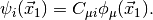Here,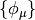are the basis functions, which, in Psi4 are contracted cartesian Gaussian functions often referred to as Atomic Orbitals (AOs). The matrix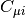contains the MO coefficients, which are the constrained variational parameters in Hartree-Fock. The molecular orbitals, are used to build the simplest possible antisymmetric wavefunction, a single Slater determinant,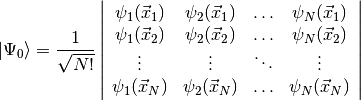This form for the Hartree-Fock wavefunction is actually entirely equivalent to treating the electron correlation as a mean field repulsion in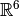instead of a more complicated effect in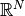.

Considering the electronic Hamiltonian,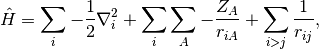the Hartree-Fock energy is, by Slater’s rules,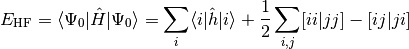Here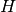is the AO-basis one-electron potential, encapsulating both electron-nuclear attraction and kinetic energy,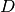is the AO-basis density matrix, build from the occupied orbital coefficients,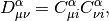and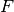is the Fock matrix, which is the effective one-body potential at the current value of the density,Here the tensor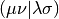is an AO Electron-Repulsion Integral (ERI) in chemists’ notation,The MO coefficients are found as the generalized eigenvectors of the Fock Matrix,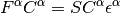The eigenvaluesare the orbital energies, and the metric matrix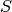is the AO-basis overlap matrixNote that the Fock Matrix depends on the density (both alpha and beta), and therefore the orbitals. Because of this, SCF is a nonlinear procedure, which terminates when the generating orbitals are self-consistent with the Fock matrix they generate.

The formation of the Coulomb matrixand the exchange matrix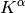dominate the computational effort of the SCF procedure. For very large systems, diagonalization of the Fock matrix can also present a significant hurdle.

Minimal Input¶

Minimal input for a Hartree-Fock computation is a molecule block, basis set option, and a call to energy('scf'):

molecule {
He
}

set basis sto-3g

energy('scf')


This will run a Restricted Hartree-Fock (RHF) on neutral singlet Helium in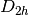spatial symmetry with a minimal STO-3G basis, 1.0E-6 energy and 1.0E-5 density convergence criteria (since single-point, see SCF Convergence & Algorithm), a DF ERI algorithm, symmetric orthogonalization, DIIS, and a core Hamiltonian guess. For more information on any of these options, see the relevant section below.

Spin/Symmetry Treatment¶

Psi4 implements the most popular spin specializations of Hartree-Fock theory, including:

Restricted Hartree-Fock (RHF) [Default]
Appropriate only for closed-shell singlet systems, but twice as efficient as the other flavors, as the alpha and beta densities are constrained to be identical.
Unrestricted Hartree-Fock (UHF)
Appropriate for most open-shell systems, and fairly easy to converge. The spatial parts of the alpha and beta orbitals are fully independent of each other, which allows a considerable amount of flexibility in the wavefunction. However, this flexibility comes at the cost of spin symmetry; UHF wavefunctions need not be eigenfunctions of the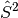operator. The deviation of this operator from its expectation value is printed on the output file. If the deviation is greater than a few hundredths, it is advisable to switch to an ROHF to avoid this “spin-contamination” problem.
Restricted Open-Shell Hartree-Fock (ROHF)
Appropriate for open-shell systems where spin-contamination is problem. Sometimes more difficult to converge, and assumes uniformly positive spin polarization (the alpha and beta doubly-occupied orbitals are identical).
Constrained Unrestricted Hartree-Fock (CUHF)
A variant of ROHF that starts from a UHF ansatz, and is therefore often easier to converge.

These can be invoked by the REFERENCE keyword, which defaults to RHF. The charge and multiplicity may either be specified in the molecule definition:

molecule h {
0 2 # Neutral doublet
H
}


or, dynamically, by setting the relevant attributes in the Python molecule object:

h.set_molecular_charge(0)
h.set_multiplicity(2)


Abelian spatial symmetry is fully supported in Psi4, and can be used to obtain physical interpretation of the molecular orbitals, to assist in difficult convergence cases, and, in some methods, to obtain significant performance gains. The point group of the molecule is inferred when reading the molecule section, and may be overridden by the symmetry flag, as in:

molecule h {
0 2
H
symmetry c1
}


or by the set_point_group Python molecule attribute:

h.set_point_group('c2v')


During the SCF procedure, the occupation of orbitals is typically determined by the Aufbau principal across all spatial symmetries. This may result in the occupation shifting between iterations. If the occupations are known a priori, they may be clamped throughout the procedure by using the DOCC and SOCC options. For instance, all good quantum chemists know that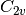water is actually,:

molecule h2o {
0 1
O
H 1 1.0
H 1 1.0 2 104.5
}

set {
docc [3,0,1,1] # 1A1 2A1 1B1 3A1 1B2
basis cc-pvdz
}

energy('scf')


Broken Symmetry¶

For certain problems, such diradicals, allowing the spin-up and spin-down orbitals to differ in closed-shell computations can be advantageous; this is known as symmetry breaking. The resulting unrestricted wavefunction will often provide superior energetics, due to the increased flexibility, but will suffer non-physicical spin contamination from higher multiplicity states. A convenient approach to break symmetry is to perform a UHF or UKS calculation with the guess HOMO and LUMO orbitals mixed. Mixing of the guess orbitals can be requested by setting the GUESS_MIX keyword to true:

set reference uhf
set guess_mix true
energy('scf')


Orthogonalization¶

One of the first steps in the SCF procedure is the determination of an orthogonal basis (known as the OSO basis) from the atomic orbital basis (known as the AO basis). The Molecular Orbital basis (MO basis) is then built as a particular unitary transformation of the OSO basis. In Psi4, the determination of the OSO basis is accomplished via either symmetric or canonical orthogonalization. Symmetric orthogonalization uses the symmetric inverse square root of the overlap matrix for the orthogonalization matrix. Use of symmetric orthogonalization always yields the same number of OSO functions (and thereby MOs) as AO functions. However, this may lead to numerical problems if the overlap matrix has small eigenvalues, which may occur for large systems or for systems where diffuse basis sets are used. This problem may be avoided by using canonical orthogonalization, in which an asymmetric inverse square root of the overlap matrix is formed, with numerical stability enhanced by the elimination of eigenvectors corresponding to very small eigenvalues. As a few combinations of AO basis functions may be discarded, the number of canonical-orthogonalized OSOs and MOs may be slightly smaller than the number of AOs. In Psi4, symmetric orthogonalization is used by default, unless the smallest overlap eigenvalue falls below the user-supplied double option S_TOLERANCE, which defaults to 1E-7. If the smallest eigenvalue is below this cutoff, canonical orthogonalization is forced, and all eigenvectors corresponding to eigenvalues below the cutoff are eliminated. Use of canonical orthogonalization can be forced by setting the S_ORTHOGONALIZATION option to CANONICAL. Note that in practice, the MOs and OSOs are built separately within each irrep from the symmetry-adapted combinations of AOs known as Unique Symmetry Orbitals (USOs). For canonical orthogonalization, this implies that the number of MOs and OSOs per irrep may be slightly smaller than the number of USOs per irrep.

A contrived example demonstrating OSOs/MOs vs. AOs with symmetry is shown below:

molecule h2o {
0 1
O
H 1 1.0
H 1 1.0 2 104.5
symmetry c2 # Two irreps is easier to comprehend
}

set {
s_tolerance 0.0001      # Set an unreasonably tight
# tolerance to force canonical
basis aug-cc-pv5z       # This diffuse basis will have
# small-ish eigenvalues for even H2O
}

energy('scf')


Output:

... Initialization ...

==> Pre-Iterations <==

Minimum eigenvalue in the overlap matrix is 1.6888059293E-05.
Using Canonical Orthogonalization with cutoff of 1.0000000000E-04.
Overall, 3 of 287 possible MOs eliminated.

... Initial Orbital Guess Information ...

-------------------------------------------------------
Irrep   Nso     Nmo     Nalpha   Nbeta   Ndocc  Nsocc
-------------------------------------------------------
A        145     144       3       3       3       0
B        142     140       2       2       2       0
-------------------------------------------------------
Total     287     284       5       5       5       0
-------------------------------------------------------


In this example, there are 287 AO basis functions after spherical harmonics are applied. These are used to produce 287 symmetry adapted USOs, 145 of which are assigned to irrep A, and 142 of which are assigned to irrep B. Within irrep A, 144 OSOs fall above the eigenvalue cutoff, and within irrep B 140 OSOs fall above the eigenvalue cutoff. In total, 284 molecular orbitals are chosen from 287 AOs/USOs. The table also shows the initial assignment of electrons to irreps.

Initial Guess/Convergence Stabilization¶

In each step of the SCF procedure, a new Fock or Kohn–Sham potential is built according to the previous density, following which the potential is diagonalized to produce new molecular orbitals, from which a new density is computed. This procedure is continued until either convergence is reached or a preset maximum number of iterations is exceeded. Convergence is determined by both change in energy and root-mean-square change in density matrix values, which must be below the user-specified E_CONVERGENCE and D_CONVERGENCE, respectively. The maximum number of iterations is specified by the MAXITER option. It should be noted that SCF is a chaotic process, and, as such, often requires careful selection of initial orbitals and damping during iterations to ensure convergence. This is particularly likely for large systems, metallic systems, multireference systems, open-shell systems, anions, and systems with diffuse basis sets.

For initial orbital selection, several options are available. These include:

CORE [Default]
Diagonalization of the core Hamiltonian, removing even mean-field electron repulsion. Simple, but often too far from the final solution for larger systems. READ becomes the default for the second and later iterations of geometry optimizations.
Superposition of Atomic Densities. Builds the initial density as the spin-averaged sum of atomic UHF computations in the current basis. If an open-shell system, uniform scaling of the spin-averaged density matrices is performed. If orbitals are needed (e.g., in density fitting), a partial Cholesky factorization of the density matrices is used. Often extremely accurate, particularly for closed-shell systems.
GWH
Generalized Wolfsberg-Helmholtz, a simple Huckel-Theory-like method based on the overlap and core Hamiltonian matrices. May be useful in open-shell systems.
Read the previous orbitals from a checkpoint file, casting from one basis to another if needed. Useful for starting anion computations from neutral orbitals, or after small geometry changes. At present, casting from a different molecular point group is not supported. This becomes the default for the second and later iterations of geometry optimizations.

These are all set by the GUESS keyword. Also, an automatic Python procedure has been developed for converging the SCF in a small basis, and then casting up to the true basis. This can be done by adding BASIS_GUESS = SMALL_BASIS to the options list. We recommend the 3-21G basis for the small basis due to its efficient mix of flexibility and compactness. An example of performing an RHF solution of water by SAD guessing in a 3-21G basis and then casting up to cc-pVTZ is shown below:

molecule h2o {
0 1
O
H 1 1.0
H 1 1.0 2 104.5
}

set {
basis cc-pvtz
basis_guess 3-21G
}

energy('scf')


With regard to convergence stabilization, Pulay’s Direct Inversion of the Iterative Subspace (DIIS) extrapolation, Gill’s Maximum Overlap Method (MOM), and damping are all implemented. A summary of each is presented below,

DIIS [On by Default]
DIIS uses previous iterates of the Fock Matrix together with an error criterion based on the orbital gradient to produce an informed estimate of the next Fock Matrix. DIIS is almost always necessary to converge the SCF procedure and is therefore turned on by default. In rare cases, the DIIS algorithm may need to be modified or turned off altogether, which may be accomplished via the options detailed below.
MOM [Off by Default]
MOM was developed to combat a particular class of convergence failure: occupation flipping. In some cases, midway though the SCF procedure, a partially converged orbital which should be occupied in the fully-optimized SCF solution has a slightly higher orbital eigenvalue than some other orbital which should be destined to be a virtual orbital. This results in the virtual orbital being spuriously occupied for one or more iterations. Sometimes this resolves itself without help, other times the occupation flips back and forth between two, four, or more orbitals. This is typically visible in the output as a non-converging SCF which eventually settles down to steady oscillation between two (or more) different total energies. This behavior can be ameliorated by choosing occupied orbitals by “shape” instead of by orbital eigenvalue, i.e., by choosing the set of new orbitals which looks most like some previously known “good” set. The “good” set is typically the occupied orbitals from an one of the oscillating iterations with the lowest total energy. For an oscillating system where the lowest total energy occurs on iterations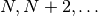, invoking MOM_START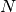can often rescue the convergence of the SCF. MOM can be used in concert with DIIS, though care should be taken to not turn MOM on until the oscillatory behavior begins.
Damping [Off by Default]
In some cases, a static mixing of Fock Matrices from adjacent iterations can quench oscillations. This mixing, known as “damping” can be activated by setting the DAMPING_PERCENTAGE keyword to a nonzero percent.

ERI Algorithms¶

The key difficulty in the SCF procedure is treatment of the four-index ERI contributions to the Fock Matrix. A number of algorithms are available in Psi4 for these terms. The algorithm is selected by the SCF_TYPE keyword, which may be one of the following

PK [Default]
An out-of-core, presorted algorithm using exact ERIs. Quite fast for a zero-error algorithm if enough memory is available. Integrals are generated only once, and symmetry is utilized to reduce number of integrals.
OUT_OF_CORE
An out-of-core, unsorted algorithm using exact ERIs. Overcomes the memory bottleneck of the current PK algorithm. Integrals are generated only once, and symmetry is utilized to reduce number of integrals.
DIRECT
A threaded, sieved, integral-direct algorithm, with full permutational symmetry. This algorithm is brand new, but seems to be reasonably fast up to 1500 basis function, uses zero disk, and can obtain significant speedups with negligible error loss if the INTS_TOLERANCE value is set to 1.0E-8 or so.
DF [Default]
A density-fitted algorithm designed for computations with thousands of basis functions. This algorithm is highly optimized, and is threaded with a mixture of parallel BLAS and OpenMP. Note that this algorithm should use the -JKFIT series of auxiliary bases, not the -RI or -MP2FIT bases. The default guess for auxiliary basis set should work for all Dunning bases, otherwise the DF_BASIS_SCF keyword can be used to manually specify the auxiliary basis. This algorithm is preferred unless either absolute accuracy is required [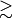CCSD(T)] or a -JKFIT auxiliary basis is unavailable for the primary basis/atoms involved.
CD
A threaded algorithm using approximate ERI’s obtained by Cholesky decomposition of the ERI tensor. The accuracy of the Cholesky decomposition is controlled by the keyword CHOLESKY_TOLERANCE. This algorithm is similar to the DF algorithm, but it is not suitable for gradient computations. The algorithm to obtain the Cholesky vectors is not designed for computations with thousands of basis functions.

For some of these algorithms, Schwarz and/or density sieving can be used to identify negligible integral contributions in extended systems. To activate sieving, set the INTS_TOLERANCE keyword to your desired cutoff (1.0E-12 is recommended for most applications).

Recently, we have added the automatic capability to use the extremely fast DF code for intermediate convergence of the orbitals, for SCF_TYPE DIRECT. At the moment, the code defaults to cc-pVDZ-JKFIT as the auxiliary basis, unless the user specifies DF_BASIS_SCF manually. For some atoms, cc-pVDZ-JKFIT is not defined, so this procedure will fail. In these cases, you will see an error message of the form:

RuntimeError: sanity check failed! Gaussian94BasisSetParser::parser:
Unable to find the basis set for HE


This failure can be fixed by either setting DF_BASIS_SCF to an auxiliary basis set defined for all atoms in the system, or by setting DF_SCF_GUESS to false, which disables this acceleration entirely.

Second-order Convergence¶

Second-order convergence takes into account both the gradient and Hessian to take a full Newton step with respect to the orbital parameters. This results in quadratic convergence with respect to density for SCF methods. For cases where normal acceleration methods either fail or take many iterations to converge, second-order can reduce the total time to solution.

Solving second-order (SO) methods exactly would require an inversion of the orbital Hessian (an expensive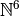operation); however, these equations are normally solved iteratively where each iteration costs the same as a normal Fock build (). The overall SOSCF operation is thus broken down into micro- and macroiterations where the microiterations refer to solving the SOSCF equations and macroiterations are the construction of a new Fock matrix based on the orbitals from a SOSCF step.

SOSCF requires that all elements of the gradient to be less than one before the method is valid. To this end, pre-SOSCF SCF iterations use normal gradient-based extrapolation procedures (e.g., DIIS) until the gradient conditions are met. Note that while the total number of macroiterations will be less for SOSCF than gradient-based convergence acceleration the cost of solving the microiterations typically results in the overall cost being greater for SOSCF than for gradient-based methods. Therefore, SOSCF should only be used if it is difficult to locate a stable minimum.

SOSCF is only available for RHF, ROHF, and UHF reference. To turn on simply set the option SOSCF to true. Additional options to modify the number of microiterations taken are as follows:

SOSCF_R_START: when to start SOSCF based on the current density RMS

SOSCF_MAX_ITER: the maximum number of SOSCF microiterations per macroiteration

SOSCF_CONV: the relative convergence tolerance of the SOSCF microiterations

SOSCF_PRINT: option to print the microiterations or not

Stability Analysis¶

SCF algorithms attempt to minimize the gradient of the energy with respect to orbital variation parameters. At convergence, the gradient should be approximately zero given a convergence criterion. Although this is enough to make sure the SCF converged to a stationary point, this is not a sufficient condition for a minimal SCF solution. It may be a sadle point or a maximum.

To ensure that a minimum has been found, the electronic Hessian, i.e. the matrix of second derivatives of the energy with respect to orbital variation parameters, must be computed. If one or more eigenvalues of the electronic Hessian are negative, the SCF solution is not a minimum. In that case, orbital parameters can be varied along the lowest Hessian eigenvector to lower the energy.

Orbital variation parameters are usually constrained. For example, in RHF the spatial parts of the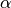and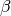orbitals are the same. In UHF, the orbital coefficients are usually constrained to be real. A stability analysis can check whether a lower SCF solution exists while respecting the constraints of the original solution; this is an internal instability. If one or more constraints have to be relaxed to reach a lower-energy solution, there is an external instability. In Psi4, the only external instability that can be checked at present is the RHF->UHF one.

Currently, two algorithms exist in Psi4 for stability analysis: the original Direct Inversion and the newly implemented Davidson algorithms. We will first describe options common to both algorithms. To request a stability analysis at the end of the SCF, set the keyword STABILITY_ANALYSIS:

set stability_analysis check


to only compute the electronic Hessian eigenvalue and check if an actual SCF minimum has been found, or:

set stability_analysis follow


to rotate the converged orbitals along the lowest eigenvector, then invoke the SCF procedure again to lower the energy. In case the minimization does not succeed or ends up on the same unstable solution, you can tune the scale factor for the orbital rotation through the keyword FOLLOW_STEP_SCALE:

set follow_step_scale 0.5


The rotation angle is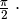(FOLLOW_STEP_SCALE). The default value of 0.5 usually provides a good guess and modification is only recommended in difficult cases. The default behavior for the stability code is to stop after trying to reoptimize the orbitals once if the instability still exists. For more attempts, set MAX_ATTEMPTS:

set max_attempts 1


the default value of 1 is recommended. In case the SCF ends up in the same minimum, modification of FOLLOW_STEP_SCALE is recommended over increasing MAX_ATTEMPTS.

The main algorithm available in Psi4 is the Direct Inversion algorithm. It can only work with SCF_TYPE PK, and it explicitly builds the full electronic Hessian matrix before explicitly inverting it. As such, this algorithm is very slow and it should be avoided whenever possible. Direct Inversion is automatically invoked if the newer algorithm is not available.

The Davidson algorithm for stability analysis was implemented recently. Only the lowest eigenvalues of the electronic Hessian are computed, and Hessian-vector products are computed instead of the full Hessian. This algorithm is thus much more efficient than the Direct Inversion, but is at present only available for UHF->UHF stability analysis. The capabilities of both algorithms are summarized below:

Stability analysis methods available in Psi4
Algorithm REFERENCE Stability checked SCF_TYPE
Direct Inversion RHF Internal, External (->UHF) PK only
ROHF Internal PK only
Davidson UHF Internal Anything

The best algorithm is automatically selected, i.e. Davidson for UHF->UHF and Direct Inversion otherwise.

In addition to the options available for Direct Inversion, the Davidson algorithm can automatically adapt FOLLOW_STEP_SCALE to find a new SCF minimum. If MAX_ATTEMPTS > 1, additional attempts will automatically increment FOLLOW_STEP_SCALE by 0.2 every time the SCF falls back to the previously found unstable minimum. The increment can be adjusted by setting FOLLOW_STEP_INCREMENT:

set follow_step_increment 0.2


The default value is 0.2, adjust if needed to try different values of FOLLOW_STEP_SCALE in a single compution.

The Davidson solver for the eigenvalues is controlled through several keywords. In the following we only report the most pertinent for stability analysis, see documentation for the CPHF module for a complete list. Some default values were modified for the stability analysis code, in that case they are explicitly indicated here.

SOLVER_MAXITER: maximum number of iterations

SOLVER_CONVERGENCE: eigenvector convergence threshold

SOLVER_N_ROOT: Solve for N eigenvectors in each irreducible representation

SOLVER_N_GUESS: Use N guess vectors, this needs to be larger than the number of roots so that the lowest ones can be captured reliably. Default within this context: 3

SOLVER_MIN_SUBSPACE: Minimum size of the subspace when collapsing.

SOLVER_MAX_SUBSPACE: Maximum size of the subspace. Default within this context: 12

In case convergence problems are encountered during the Davidson procedure, it is recommended to first increase SOLVER_MAX_SUBSPACE, especially if you solve for a large number of roots. This will result in a higher computational cost of each iteration, but should make the solver better behaved. However, note that SOLVER_MAX_SUBSPACE should never be larger than the full subspace minus the number of desired roots to avoid adding artificial zero eigenvalues. This may happen in minimal basis sets, especially with symmetry, but the code automatically adjusts SOLVER_MAX_SUBSPACE if it is too large. If the solver seems to converge on the wrong eigenvalue, try increasing SOLVER_N_GUESS. Otherwise, if the solver is almost converged but reaches the maximum number of iterations, try increasing SOLVER_MAXITER.

External potentials and QM/MM¶

In addition to the implementation of EFP for accurate QM/MM computations, Psi4 can perform more rudimentary QM/MM procedures via the EXTERN keyword. The following snippet, extracted from the extern1 test case, demonstrates its use for a TIP3P external potential:

Chrgfield = QMMM()
psi4.set_global_option_python('EXTERN',Chrgfield.extern)


First a QMMM object is created, then three separate particles are added to this object before the SCF code is told about its existence on the last line. The calls to addCharge take the atomic charge, x coordinate, y coordinate, and z coordinate in that order. The atomic charge is specified in atomic units, and the coordinates always use the same units as the geometry specification in the regular QM region. Additional MM molecules may be specified by adding extra calls to addCharge to describe the full MM region.

Convergence and Algorithm Defaults¶

SCF algorithm and convergence criteria defaults by calculation type 
Ab Initio Method Calculation Type E_CONVERGENCE D_CONVERGENCE SCF_TYPE
SCF of HF or DFT energy 6 6 DF
optimization 8 8
frequency  8 8
SCF of post-HF energy 8 8 PK 
optimization 10 10
frequency  10 10
CC property  10 10
Post-SCF convergence criteria defaults by calculation type 
Ab Initio Method Calculation Type E_CONVERGENCE  R_CONVERGENCE 
post-HF of post-HF energy 6
optimization 8
frequency  8
CC property  8

Footnotes

  Note that this table applies only the SCF module, not to the final convergence criteria for post-HF methods or to methods that use an alternate starting point, like MCSCF. SAPT computations, too, set tighter values.
  (1, 2) This applies to properties computed through the property() function.
  Post-HF methods that do not rely upon the usual 4-index AO integrals use a density- fitted SCF reference. That is, for DF-MP2 and SAPT, the default SCF_TYPE is DF.
  Note that this table applies to the final convergence criteria for all the post-SCF modules that define a E_CONVERGENCE keyword.
  The E_CONVERGENCE keyword is implemented for most post-SCF modules. See a list beginning at E_CONVERGENCE.
  The R_CONVERGENCE keyword places a convergence check on an internal residual error measure and is implemented for several post-SCF modules (see list beginning at R_CONVERGENCE). It is defined according to the quantum chemical method and so its default value is set by each module individually.
  (1, 2, 3) For frequency computations by finite difference of energies, convergence criteria are tightened further still to 10 for E_CONVERGENCE and D_CONVERGENCE for SCF of HF or DFT, 11 for E_CONVERGENCE and D_CONVERGENCE for SCF of post-HF, and 10 for E_CONVERGENCE for post-HF of post-HF.

Recommendations¶

The SCF code is already quite flexible and powerful, with new features being added weekly. We have tried as much as possible to keep the number of options to a minimum, and to allow all options to be used in the presence of all other options. Below are some rough words of advice about using the SCF code for practical calculations:

• For GUESS, the SAD guess is usually your friend, even for open-shell systems (at the very least, it gets the right number of electrons, unlike some other programs). For instance, we have found that a simple SAD guess is often as good as doing a full SCF in a 3-21G basis and then performing a cast-up, at a fraction of the cost. However, SAD and DOCC/SOCC arrays do not play very well together at the moment. Also, the SAD UHF guess is very slow in large basis sets, so you may want to cast up for >TZ.
• For wall time, DF may be a factor of ten or more faster than the exact integral technologies available in PSI4. Use DF unless you need absolute accuracy or do not have a -JKFIT auxiliary set for your primary basis/atom type. Then use DIRECT.
• Don’t mess with the DIIS convergence options unless convergence is a problem. We have optimized the parameters for efficiency over a wide array of system types.
• Buy a developer a beer!

The “best-practice” input file for HF is:

memory 1 GB # As much as you've got, the DF algorithm can use

molecule {
O
H 1 1.0
H 1 1.0 2 104.5
}

set {
basis cc-pvdz
scf_type df